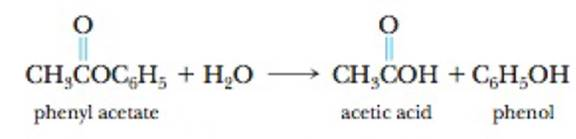# Phenyl acetate, an ester, reacts with water according to the equation The data in the table were collected for this reaction at 5°C. Time (s) [Phenyl acetate] (mol/L) 0 0.55 15.0 0.42 30.0 0.31 45.0 0.23 60.0 0.17 75.0 0.12 90.0 0.085 (a) Plot the phenyl acetate concentration versus time, and describe the shape of the curve observed. (b) Calculate the rate of change of the phenyl acetate concentration during the period 15.0 seconds to 30.0 seconds and also during the period 75.0 seconds to 90.0 seconds. Why is one value smaller than the other? (c) What is the rate of change of the phenyl acetate concentration during the time period 60.0 seconds to 75.0 seconds? (d) What is the instantaneous rate at 15.0 seconds?### Chemistry & Chemical Reactivity

9th Edition
John C. Kotz + 3 others
Publisher: Cengage Learning
ISBN: 9781133949640

#### Solutions

Chapter
Section### Chemistry & Chemical Reactivity

9th Edition
John C. Kotz + 3 others
Publisher: Cengage Learning
ISBN: 9781133949640
Chapter 14, Problem 6PS
Textbook Problem
172 views

## Phenyl acetate, an ester, reacts with water according to the equationThe data in the table were collected for this reaction at 5°C. Time (s) [Phenyl acetate] (mol/L) 0 0.55 15.0 0.42 30.0 0.31 45.0 0.23 60.0 0.17 75.0 0.12 90.0 0.085 (a) Plot the phenyl acetate concentration versus time, and describe the shape of the curve observed. (b) Calculate the rate of change of the phenyl acetate concentration during the period 15.0 seconds to 30.0 seconds and also during the period 75.0 seconds to 90.0 seconds. Why is one value smaller than the other? (c) What is the rate of change of the phenyl acetate concentration during the time period 60.0 seconds to 75.0 seconds? (d) What is the instantaneous rate at 15.0 seconds?

(a)

Interpretation Introduction

Interpretation:

The Rate change should be calculated.

Concept introduction:

Rate change of the reaction is calculated by using following formula,

Rate change =change in concentrationchangeintime

### Explanation of Solution

(a)

(b)

Interpretation Introduction

Interpretation:

The Rate change should be calculated.

Concept introduction:

Rate change of the reaction is calculated by using following formula,

Rate change =change in concentrationchangeintime

(c)

Interpretation Introduction

Interpretation:

The Rate change should be calculated.

Concept introduction:

Rate change of the reaction is calculated by using following formula,

Rate change =change in concentrationchangeintime

(d)

Interpretation Introduction

Interpretation:

The Rate change should be calculated.

Concept introduction:

Rate change of the reaction is calculated by using following formula,

Rate change =change in concentrationchangeintime

### Still sussing out bartleby?

Check out a sample textbook solution.

See a sample solution

#### The Solution to Your Study Problems

Bartleby provides explanations to thousands of textbook problems written by our experts, many with advanced degrees!

Get Started

Find more solutions based on key concepts
You are driving toward a cliff and honk your horn. Is there a Doppler shift of the sound when you hear the echo...

Physics for Scientists and Engineers, Technology Update (No access codes included)

22-8 What are the two basic classes of proteins?

Introduction to General, Organic and Biochemistry

Do heterotrophs (zooplankton) have a compensation depth?

Oceanography: An Invitation To Marine Science, Loose-leaf Versin

Alcohol is a natural substance and therefore does no real damage to body tissues. T F

Nutrition: Concepts and Controversies - Standalone book (MindTap Course List)